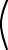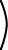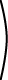A5×5 B5×3 C3×4  =  D5×4.

# Distributive Property

The distributive property deals with a matrix expression that contains both matrix multiplication and matrix addition. Go through the following steps to demonstrate the property.

1. Perform the matrix addition. Click = to check your result under step 2, below.+2. Multiply the two matrices. Click "=" to see the final result.3. Now, work the problem again in a different order. First form the product of the left matrix with each of the other two.+4. Finally, add up the two products.+Both results are the same, demonstrating that matrix multiplication is distributive over matrix addition:

A(B + C) = AB + AC

It is also true that

(X + Y)Z = XZ + YZ

For more practice, change the elements in the matrices of step 1 (only), and then work the problem again. When you make changes, replace the contents of a cell with an integer and click outside of the cell to make the change.

### QUESTION 12:

Calculate the following:``` cos w 1 0 sin w `````` x 2 1 y ```